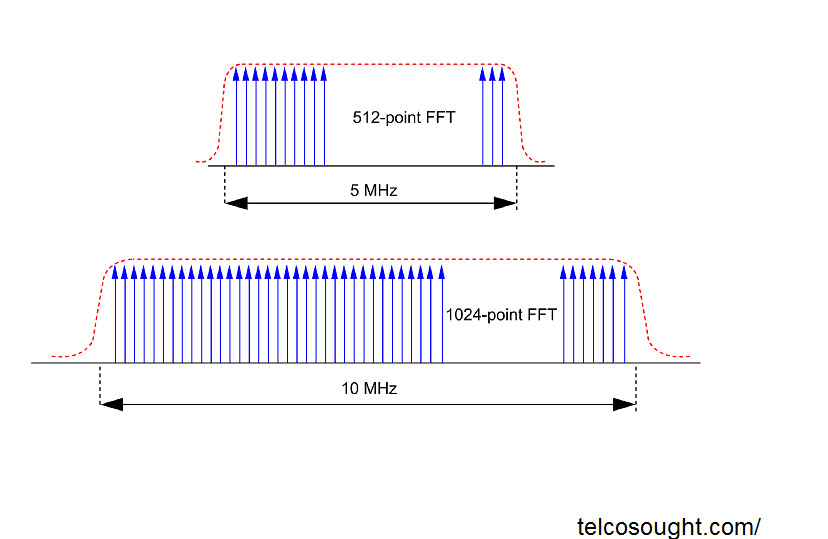# Scalability in OFDM and scalable ofdm

In this OFDM article learn about scalable scalable ofdm numerology. An OFDM technology could require to function not just in different frequency bands, but as well in various OFDM channels with different bandwidths.

## Scalability in OFDM

If the subcarriers’ number remains constant, however, that the speed of the total OFDM channel increases and the separation between subcarriers will grow in relation. The increased subcarrier separation implies that the symbol rate of each subcarrier will increase in proportion. Otherwise, any orthogonality that exists between the subcarriers would disappear.

The diagram on the top illustrates a subcarrier set that operates orthogonally.

The center diagram illustrates an example of how it is clear that the OFDM channel’s bandwidth increased, as well as the FFT size and subcarrier symbol rates, are maintained. The spacing of subcarriers has increased because of this, and it is evident that the subcarriers ‘ positions are no anymore orthogonal.

In the diagram below, the OFDM bandwidth has grown in the same proportion, but the FFT size hasn’t been altered. However, this time the symbol rate of the subcarriers has been also increased and the orthogonality has been restored.

It is therefore possible to create an OFDM system that is modified to work in varying OFDM channels with different bandwidths. However, this isn’t the most efficient way to go. The system could become more flexible, and operate better if the symbol rates on subcarriers didn’t require adjustment for various OFDM Channel bandwidths.

## Scalable OFDMIt’s been discovered that the most efficient method for working in different bandwidths is to change the size of FFT rather than using the symbol rates of subcarriers. This means that many of the important system parameters are the same for various bandwidths, which gives more chances to take advantage of channel adaption when the channel’s overall bandwidth is greater.

This method is commonly described as an OFDM that is scalable. The figure illustrates the operation of the 5 MHz frequency range that has an FFT of size 512, and it is the same system operating in a 10- millisecond channel, with the FFT of size 1024. The symbol rate and the subcarrier separation requirement is the same in both scenarios and orthogonality is preserved.

Related Post: